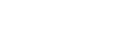## Testing

0

(click on this box to dismiss)

SELECT Reputation from Users where Reputation;Q&A for Ubuntu users and developers

``````-- Testing
-- Enter Query Description
-- SELECT Reputation from Users where Reputation;

with data as (Select count(*) as total,
(Select cast(Count(*) as decimal) from Users where SUBSTRING(CAST(Reputation AS VARCHAR), 1, 1) = '1') as one,
(Select cast(Count(*) as decimal) from Users where SUBSTRING(CAST(Reputation AS VARCHAR), 1, 1) = '2') as two,
(Select cast(Count(*) as decimal) from Users where SUBSTRING(CAST(Reputation AS VARCHAR), 1, 1) = '3') as three,
(Select cast(Count(*) as decimal) from Users where SUBSTRING(CAST(Reputation AS VARCHAR), 1, 1) = '4') as four,
(Select cast(Count(*) as decimal) from Users where SUBSTRING(CAST(Reputation AS VARCHAR), 1, 1) = '5') as five,
(Select cast(Count(*) as decimal) from Users where SUBSTRING(CAST(Reputation AS VARCHAR), 1, 1) = '6') as six,
(Select cast(Count(*) as decimal) from Users where SUBSTRING(CAST(Reputation AS VARCHAR), 1, 1) = '7') as seven,
(Select cast(Count(*) as decimal) from Users where SUBSTRING(CAST(Reputation AS VARCHAR), 1, 1) = '8') as eight,
(Select cast(Count(*) as decimal) from Users where SUBSTRING(CAST(Reputation AS VARCHAR), 1, 1) = '9') as nine
from Users ) select
one/total as "1.0",
two/total,
three/total,
four/total,
five/total,
six/total,
seven/total,
eight/total,
nine/total
from data;``````

### Enter Parameters

Options:
-Hold tight while we fetch your results
:records returned in :time ms:cached